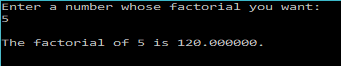# C Program to find the Factorial of a numberFactorial is defined for a positive integer, n, as the product of all the integers from 1 to n.
Factorial of n is denoted by$n!$.$n!=n \times (n-1) \times (n-2).... \times 1$
For ex: 5!=5x4x3x2x1.

To write a C program that calculates the factorial of a given integer, we will make use of for loops. We will start the loop at i=n(Integer whose factorial is required). Decrement i at every iteration by 1 until we reach i=1.

Inside the loop, we will perform the multiplications required for finding the factorial.
The C program to find the factorial of a given number(positive integer) is shown below:

### PROGRAM:

/***************************
********FACTORIAL**********
2017 (c) Manas Sharma - https://bragitoff.com
**************************/
#include<stdio.h>

/*The following function takes an int and returns it's factorial
NOTE: WE ARE USING DOUBLE AS THE RETURN TYPE TO ACCOMODATE LARGE FACTORIALS*/
double factorial(int n){
int i;
double fact=1;
for(i=n;i>=1;i--){
fact=fact*i;
}
return fact;
}

main(){
int n;
printf("Enter a number whose factorial you want:\n");
scanf("%d",&n);
printf("\nThe factorial of %d is %lf.\n\n",n,factorial(n));
}


### Output:[wpedon id="7041" align="center"]